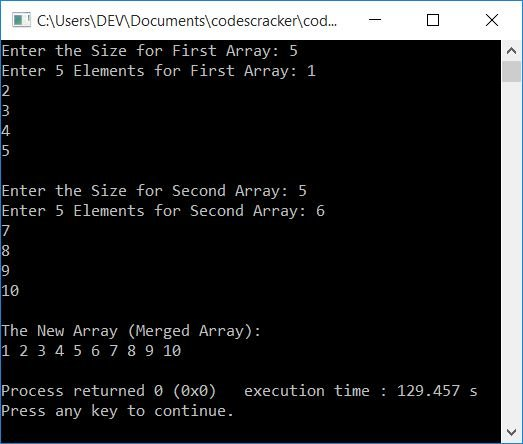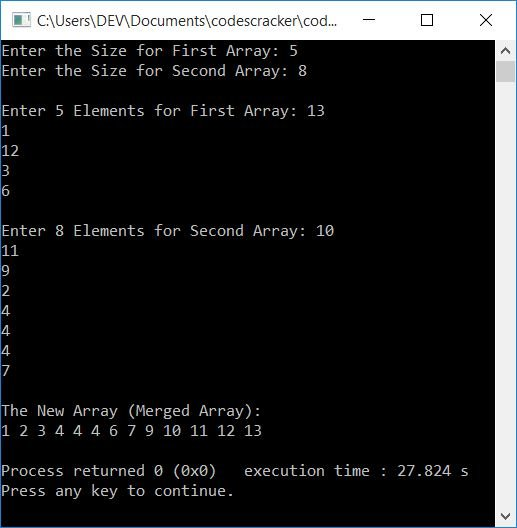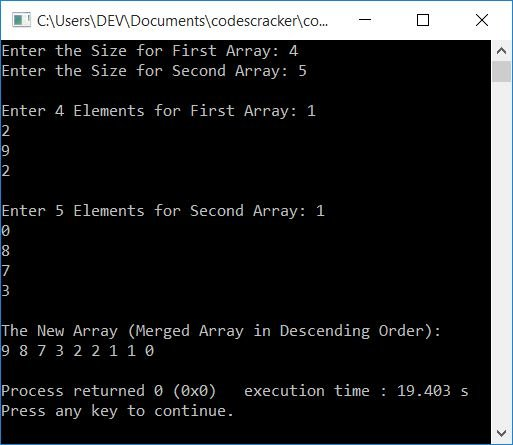# C++ Program to Merge Two Arrays

In this article, you will learn and get code to merge two arrays entered by the user at run-time in the C++ language. Here is the list of programs on the merging of two arrays available in this article:

## Merge two arrays in C++

To merge two arrays in C++ programming, you have to ask the user to enter the sizes and elements for both arrays. Then merge these two given arrays into a third array, as shown in the program given below:

Note: At the time of receiving the array's elements, we applied the merge operation. That is, the elements received from the user are added one by one to a third array.

The question is, "Write a program in C++ to merge two arrays." Here is its answer:

```#include<iostream>
using namespace std;
int main()
{
int arrOne, arrTwo, arrMerge;
int sizeOne, sizeTwo, i, k;
cout<<"Enter the Size for First Array: ";
cin>>sizeOne;
cout<<"Enter "<<sizeOne<<" Elements for First Array: ";
for(i=0; i<sizeOne; i++)
{
cin>>arrOne[i];
arrMerge[i] = arrOne[i];
}
k = i;
cout<<"\nEnter the Size for Second Array: ";
cin>>sizeTwo;
cout<<"Enter "<<sizeTwo<<" Elements for Second Array: ";
for(i=0; i<sizeTwo; i++)
{
cin>>arrTwo[i];
arrMerge[k] = arrTwo[i];
k++;
}
cout<<"\nThe New Array (Merged Array):\n";
for(i=0; i<k; i++)
cout<<arrMerge[i]<<" ";
cout<<endl;
return 0;
}```

This program was built and runs under the Code::Blocks IDE. Here is its sample run:Now supply the inputs one by one. For example, if the user enters:

• 5 as the size for the first array.
• The first array's elements are 1, 2, 3, 4, and 5.
• 5 as the second array's size.
• 6, 7, 8, 9, 10 as 5 elements for the second array.

then here is the output produced by the above program after supplying these inputs and pressing the ENTER key:You can also sort the two arrays, then merge. Alternatively, sort after merging. Here is the list of sorting techniques you can go with:

## Merge Two Arrays in Ascending Order

This program merges two arrays entered by the user at run-time in ascending order. That is, the two arrays get merged and then sorted before printing the merged array on output.

```#include<iostream>
using namespace std;
int main()
{
int arrOne, arrTwo, arrMerge;
int sizeOne, sizeTwo, sizeMerge, i, j, temp;
cout<<"Enter the Size for First Array: ";
cin>>sizeOne;
cout<<"Enter the Size for Second Array: ";
cin>>sizeTwo;
cout<<"\nEnter "<<sizeOne<<" Elements for First Array: ";
for(i=0; i<sizeOne; i++)
cin>>arrOne[i];
cout<<"\nEnter "<<sizeTwo<<" Elements for Second Array: ";
for(i=0; i<sizeTwo; i++)
cin>>arrTwo[i];
// merging the two arrays
for(i=0; i<sizeOne; i++)
{
arrMerge[i] = arrOne[i];
}
for(j=0; j<sizeTwo; j++)
{
arrMerge[i] = arrTwo[j];
i++;
}
sizeMerge = i;
// sorting the merged array in ascending order
for(j=0; j<(sizeMerge-1); j++)
{
for(i=0; i<(sizeMerge-1); i++)
{
if(arrMerge[i]>arrMerge[i+1])
{
temp = arrMerge[i];
arrMerge[i] = arrMerge[i+1];
arrMerge[i+1] = temp;
}
}
}
cout<<"\nThe New Array (Merged Array):\n";
for(i=0; i<sizeMerge; i++)
{
if(i==(sizeMerge-1))
cout<<arrMerge[i];
else
cout<<arrMerge[i]<<" ";
}
cout<<endl;
return 0;
}```

Here is its sample run, with the following user inputs:

• 5 as the size of the first array
• 8 as the size of the second array
• 13, 1, 12, 3, 6, as 5 elements for the first array
• The second array has 8 elements: 10, 11, 9, 2, 4, 4, 4, 7.

After supplying these inputs, here is the output produced by the above program:## Merge two arrays in descending order

To merge two arrays in descending order, change the following code (from the previous program):

`if(arrMerge[i]>arrMerge[i+1])`

with

`if(arrMerge[i]<arrMerge[i+1])`

It's worth noting that changing the greater-than and less-than symbols changes the entire program.

Everything else will remain the same.That is, the code that sorted merged arrays in ascending order in the previous program has changed to sort the same arrays in descending order.

But still, if you want the complete program on the merging of two arrays in descending order, here is the source code for you:

```#include<iostream>
using namespace std;
int main()
{
int arrOne, arrTwo, arrMerge;
int sizeOne, sizeTwo, sizeMerge, i, j, temp;
cout<<"Enter the Size for First Array: ";
cin>>sizeOne;
cout<<"Enter the Size for Second Array: ";
cin>>sizeTwo;
cout<<"\nEnter "<<sizeOne<<" Elements for First Array: ";
for(i=0; i<sizeOne; i++)
cin>>arrOne[i];
cout<<"\nEnter "<<sizeTwo<<" Elements for Second Array: ";
for(i=0; i<sizeTwo; i++)
cin>>arrTwo[i];
// merging the two arrays
for(i=0; i<sizeOne; i++)
{
arrMerge[i] = arrOne[i];
}
for(j=0; j<sizeTwo; j++)
{
arrMerge[i] = arrTwo[j];
i++;
}
sizeMerge = i;
// sorting the merged array in descending order
for(j=0; j<(sizeMerge-1); j++)
{
for(i=0; i<(sizeMerge-1); i++)
{
if(arrMerge[i]<arrMerge[i+1])
{
temp = arrMerge[i];
arrMerge[i] = arrMerge[i+1];
arrMerge[i+1] = temp;
}
}
}
cout<<"\nThe New Array (Merged Array in Descending Order):\n";
for(i=0; i<sizeMerge; i++)
{
if(i==(sizeMerge-1))
cout<<arrMerge[i];
else
cout<<arrMerge[i]<<" ";
}
cout<<endl;
return 0;
}```

Here is its sample run with the following user inputs:

• 4 as the size of the first array
• The size of the second array is set to 5.
• 1, 2, 9, 2 as 4 elements for the first array
• 1, 0, 8, 7, 3 as 5 elements for the second array

The snapshot given below shows the sample output produced by the above program after supplying these inputs:#### The same program in different languages

C++ Quiz

« Previous Program Next Program »